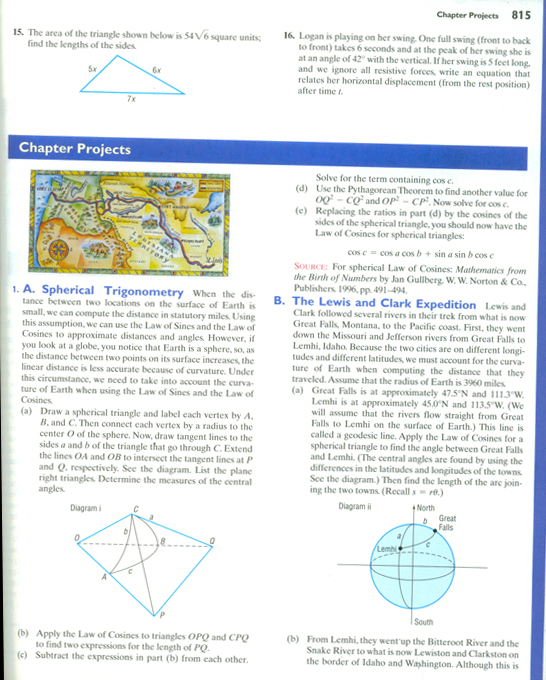_______
20090804 begin

The following is just the result of a brainstorming session to inspire ideas in other constructors. It is incomplete, but is what I am working on now. In fact it may not even work. But that is ok, because you learn by experimentation and asking questions.

The picture is a chapter problem excerpt from “Trigonometry: Enhanced with Graphing Utilities” by Michael Sullivan on page 815. I have not done the standard, textbook solution. But as long as the curve we are working with is spherical there are some very interesting and important observations we can make.

These observations may work for any defined curve. But here we are interested in a sphere, because any distance drawn along the sphere will be a circular arch. We can use what is already known about circular functions such as the sine and cosine and apply it to the sphere.

There is a distance from a radius on the unit circle between 2 radiuses where the distance is the length from the orthogonal (or perpendicular from one of the radiuses) to the new position of the 2nd radius. I call it the Ssine or Scosine. Click here to review.

This length is the change of “extra length” that a line would “fit” drawn along a sphere.

The same principle would hold true for a parabola that has a rate of change equal to that of a changing sphere. For example, from the apex draw a line at the same angle of the line on the sphere. This new line on the parabola, from the apex to where it crosses the curve of the parabola is the “extra distance due to the spherical curve”.

There may even be applications with a logarithmic spiral to measure a line that encircles the sphere many times. But again I haven’t yet proven this mathematically. It is just an idea in a brainstorming session.
_______
20090804 end

_______
20090907

This might make it little more difficult to understand the concept I am trying to convey, but the above work is where I started to do the write up of my ideas. When I wrote it, I noticed many “gaps” in the logic and truthfulness. Again let me stress that this is just a brainstorm and not at all complete. What follows is an outline of ideas that I am currently working on. I hope by reading it this brainstorm session will inspire many ideas in everyone.

This usefulness of the Scosine and Ssine in this application is questionable. But I think it just might help. The problem occurs when the Scosine or Ssine approach infinity. To make it useful we would have to represent infinity by reaching 1 or the [2 * the radius of the given sphere]. So if we were to take [ ((1 \ (value of the Ssine)) * r) + r] , we would have the length of a radius on a 3D sphere.

This prevents us from having to use the Scosine for angles less than 45 degrees and Ssine for angles greater that 45. Instead we can use the above equation to solve for radius on a 3D sphere.

There is also the problem of the circle encompassing the 3D sphere to be less than the radius of the sphere. For example are the lines of latitude on the globe near the poles that are shorter than the equator. So with this approach we would have to solve for the radius of the encompassing circle.

This radius along a 3D sphere has been solved before on Constructor’s Corner. As you may recall the "arched doorway problem” We could simply find radius of arch along the 3D sphere and use that radius in the equation [segment = angle in radians * radius] . We could subtract this result by the length of the straight line distance and get the length that was added by the 3D sphere.

Now I am going to relate the 3D spherical trigonometry to a parabola. And also relate it to the Prime Number spiral. If the apex of a parabola was drawing so that a change in apex to the curved line that forms the parabola, the apex line would represent the increase of length due to the extra length on a 3D sphere. Of course it would also show the increase of the sphere’s radius versus the increase of line (segment) on the sphere. If such a parabola could be drawn, it would add some proof to a pattern of Prime numbers along a parabola.

This work is incomplete but will be an ongoing project on Constructor’s Corner. That is if the theories have any merit.

Live long and prosper exponentially